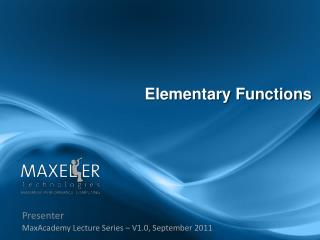DownloadDownload PresentationPresenter

# Presenter

Download Presentation## Presenter

- - - - - - - - - - - - - - - - - - - - - - - - - - - E N D - - - - - - - - - - - - - - - - - - - - - - - - - - -
##### Presentation Transcript

1. Presenter MaxAcademy Lecture Series – V1.0, September 2011 Elementary Functions

2. Lecture Overview • Motivation • How to evaluate functions • Polynomial and rational approximation • Table-based methods • Shift and add methods

3. Motivation • Elementary function are required for compute intensive applications, for example: • 2D/3D graphics: trigonometric functions • Image Processing: e.g. Gamma Function • Signal Processing, e.g. Fourier Transform • Speech input/output • Computer Aided Design (CAD): geometry calculations • and of course Scientific Applications: • Physics, Biology, Chemistry, etc…

4. Evaluating Functions • 3 steps to compute f(x) • Given argument x, find x’=g(x) with x’ in [a,b], and f(x) = h( f( g(x) )) • Step 1: Argument Reduction = g(x) • Step 2: Approximation over interval [a,b] I.e. compute f( g(x) ) • Step 3: Reconstruction: f(x) = h( f(g(x) ))

5. Example: sin(x) • Example: sin(float x) float sin(float x){ float y = x mod (π/2); // reduction float r1 = c0*y*y+c1*y+c2; float r2 = c3*y*y+c4*y+c5; return (r1/r2); // rational approx. } c0-c5 are coefficients of a rational approximation of sin(x) in [0, π/2 ]. (note: no reconstruction is needed)

6. Example f(x) = exp(x) • x / (0.5 ln 2) = N + r/(0.5 ln 2) • x = N (0.5 ln 2) + r • exp(x) = 2^ (0.5 N) *exp(r) • Step 1: • N = integer quotient of x/(0.5 ln 2) • r = remainder of x/(0.5 ln 2) • Step 2: • Compute exp(r) by approximation (e.g. polynomial) • Step 3: • Compute exp(x) = 2^ (0.5 N) *exp(r) which is just a shift!!

7. 2nd Step: Approximations in [a,b] • Polynomial and rational approximations • 1 full lookup table • Bipartite tables (2 tables + 1 add/sub) • Piecewise affine approximation (tables + mult/add) • Shift-and-add methods (with small tables)

8. Evaluating Polynomials • Horner Rule transforms polynomial into a “Multiply-Add Structure” • As a consequence, DSP Microprocessors have a Multiply-Add Instruction (Madd) by simply adding another row to an array multiplier.

9. Polynomial and Rational Approximation “Rational Approximation” “Polynomial Approximation”

10. Finding the Coefficients • Taylor series finds optimal coefficient for a specific point x=x0. • We need optimal coefficient for an entire interval [a,b]. Software such as Maple computes optimal coefficients for polynomial and rational approximations with Remez’s method (a.k.a. minimax coefficients). • Bottom line: we can find optimal coefficients for any function and any interval [a,b].

11. Table-based Methods • Full table lookup: N-bit input, M-bit output • Lookup Table Size = M2N bits • Delay of a lookup in large tables increases with size! • For N > 8 bits we need to use smaller tables: • Add elementary operations to reduce table size • Tables + 1 Add/Sub • Tables + Multiply • Tables + Multiply-Add • Tables + Shift-and-Add

12. Bi-Partite Tables x0 x1 x2 n0 n1 n2 Table a0 (x0 ,x1) Table a1 (x0 ,x2) p0 p1 Adder p ̃̃ f(x)

13. SymmetricBipartite Tables Sizes

14. Table + Multiply Add • f(x) = ax+b with a,b stored in tables • Xm are leading bits of X which determine which linear piece of f(x) should be used. TABLE xm MultAdd f(x) x

15. Shift-and-Add Methods • Fixed shift in Hardware = shifted wiring  no cost • Fixed shift = multiply by 2x • Modify Multiply-Add algorithms to only multiply by powers of 2. • Is this possible ? How do we choose the k’s, c’s?

16. CORDIC • Iterations: • e(i) = table lookup • μ = {-1,0,1} • di = ±sign(z(i)) x add/sub y constant add z 0 Parallel CORDIC

17. CORDIC on Xilinx XC4000 { X’ , Y’ } X’ X Y’ Y

18. Area-Time Tradeoff • In general we trade area for speed. Tables+Add/Sub Tables + Mult-Add Shift-and-Add small fast

19. Summary • 3 steps to compute f(x) • Step 1: Argument Reduction = g(x) • Step 2: Approximation over interval [a,b] • Lookup Table for a small number of bits. • Lookup Table + Add/Sub => Bi-partite tables • Lookup Table + Mult-Add => Piecewise Linear Approx. • Shift-and-Add Methods => e.g. CORDIC • Polynomial and Rational Approximations • Step 3: Reconstruction = h(x)

20. Further Reading on Function Evaluation • J.M. Muller, “Elementary Functions,” Birkhaeuser, Boston, 1997. • Story, S. and Tang, P.T.P., "New algorithms for improved transcendental functions on IA-64," in Proceedings of 14th IEEE symposium on computer arithmetic, IEEE Computer Society Press, 1999. • D.E. Knuth, “The Art of Computer Programming”, Vol 2, Seminumerical Algorithms, Addison-Wesley, Reading, Mass., 1969. • C.T. Fike, “Computer evaluation of mathematical functions,” Englewood Cliffs, N.J., Prentice-Hall, 1968. • L.A.Lyusternik, “Handbook for computing elementary functions”, available in english translation.

21. Exercises • Write a MaxCompiler kernel which takes an input stream x and computes a polynomial approximation of sin(x). Draw the dataflow graph. • Write a MaxCompiler kernel that implements a CORDIC block. Vary the number of stages in the CORDIC and evaluate the impact on the result.